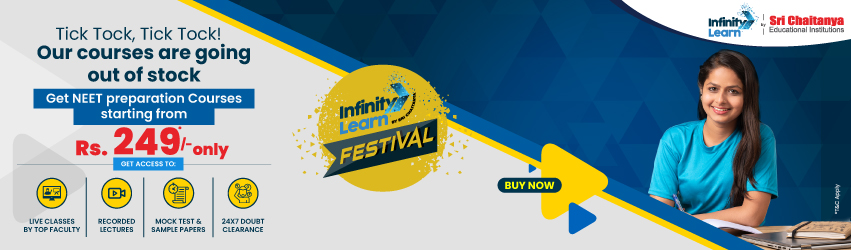NCERT Solutions for Class 11 Maths Chapter 6 Linear Inequalities Exercise 6.2

# NCERT Solutions for Class 11 Maths Chapter 6 Linear Inequalities Exercise 6.2Infinity Learn NEET Festival
Infinity Learn IIT JEE Festival

All 6.2 questions are answered in NCERT Solutions for Class 11 Maths Chapter 6 6.2 Linear Inequalities. The principals studied in Maths chapter 11 are the foundation for NCERT Solutions for Class 11 6.2. This practice is crucial for both the CBSE Term II exam and competitive exams. To do well on your exam, download these NCERT Solutions and practice them offline.

Need FREE NCERT/CBSE/IIT-JEE/NEET Study Material?

SOLUTIONS

NCERT Solutions for Class 11 Maths Chapter 6 Linear Inequalities 6.2

Using the NCERT solutions for 6.2 Class 11 Maths is the best option for the CBSE students when they are preparing for their exams. This chapter includes many exercises. One of them is Exercise 6.2 whose solutions are available in pdf format on this page. You can also download these solutions online from our website or app. Infinity Learn subject matter experts have designed solutions from the exercise with the utmost care and by keeping in mind all the guidelines by CBSE. Class 11 Students which have prepared with all the exercises given in this chapter can easily score well in the exams. By using these solutions, students came to know about the pattern of questions that are asked in the exam from this chapter and also know about the marks weightage of the chapter. So they can prepare well.

Along with this exercise, there are many number of exercises included in this chapter that contain innumerable questions as well. All these solutions are designed by subject matter experts as mentioned earlier. Hence all of these are considered as superior quality and students must refer to these solutions. To score well in the exam all these solutions must be practiced well and all other questions must be solved which are given next to it. Do not delay any more. Download the NCERT solutions for Class 11 Maths Chapter 6 6.2 from Infinity Learn website now for better exam preparation. If you have the Infinity Learn app in your phone, you should download the same through the app as well. You can use these solutions online and offline as well

1. How to express linear inequalities in two variables?

Ans. It should be expressed as ax + by < c or ax + by ≤ c or ax + by > c or ax + by ≥ c. if students need more help like this, then choose Infinity Learn best NCERT Solutions. You can download and study from these NCERT Solutions now. By using this solution students can improve their performance in examinations and tests.

2. What are the important concepts to read to complete Class 11 Maths Chapter 6?

Ans. The basics students can study from Class 11 Maths Chapter 6 are understanding inequalities and linear inequalities, Linear Inequalities’ Algebraic Solutions in one variable and two variables and their graphical representation. NCERT Solutions of this chapter on infinite Learn will help students to understand this chapter easily and can solve the exercises.

3. On which website can we get the most reliable content of this chapter?

Ans. The most reliable and accurate NCERT Solutions of this chapter are present on Infinity Learn. Students can download these solutions for free if they already have a registration. These solutions are designed and compiled by subject-matter experts having many years of experience in handling the CBSE syllabus and therefore brings the most advanced and understandable solutions to the exercises.

Join Infinity Learn Regular Class Programme!